深度学习都是非凸问题_深度学习非凸优化问题的w1 w2 - CSDN

• ## 凸优化和非凸优化

千次阅读 2018-10-09 18:59:33
是闭合的凸集且是上的函数的最优化问题，这两个条件任一不满足则该问题即为非的最优化问题。   其中，是 凸集是指对集合中的任意两点，有，即任意两点的连线段在集合内，直观上就是集合不会像下图那样有...


数学中最优化问题的一般表述是求取，使，其中是n维向量，是的可行域，是上的实值函数。

凸优化问题是指：是闭合的凸集且是上的凸函数的最优化问题，这两个条件任一不满足则该问题即为非凸的最优化问题。

其中，是 凸集是指对集合中的任意两点，有，即任意两点的连线段都在集合内，直观上就是集合不会像下图那样有“凹下去”的部分。

至于闭合的凸集，则涉及到闭集的定义，而闭集的定义又基于开集，比较抽象，不赘

述，这里可以简单地认为闭合的凸集是指包含有所有边界点的凸集。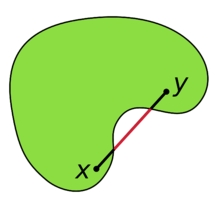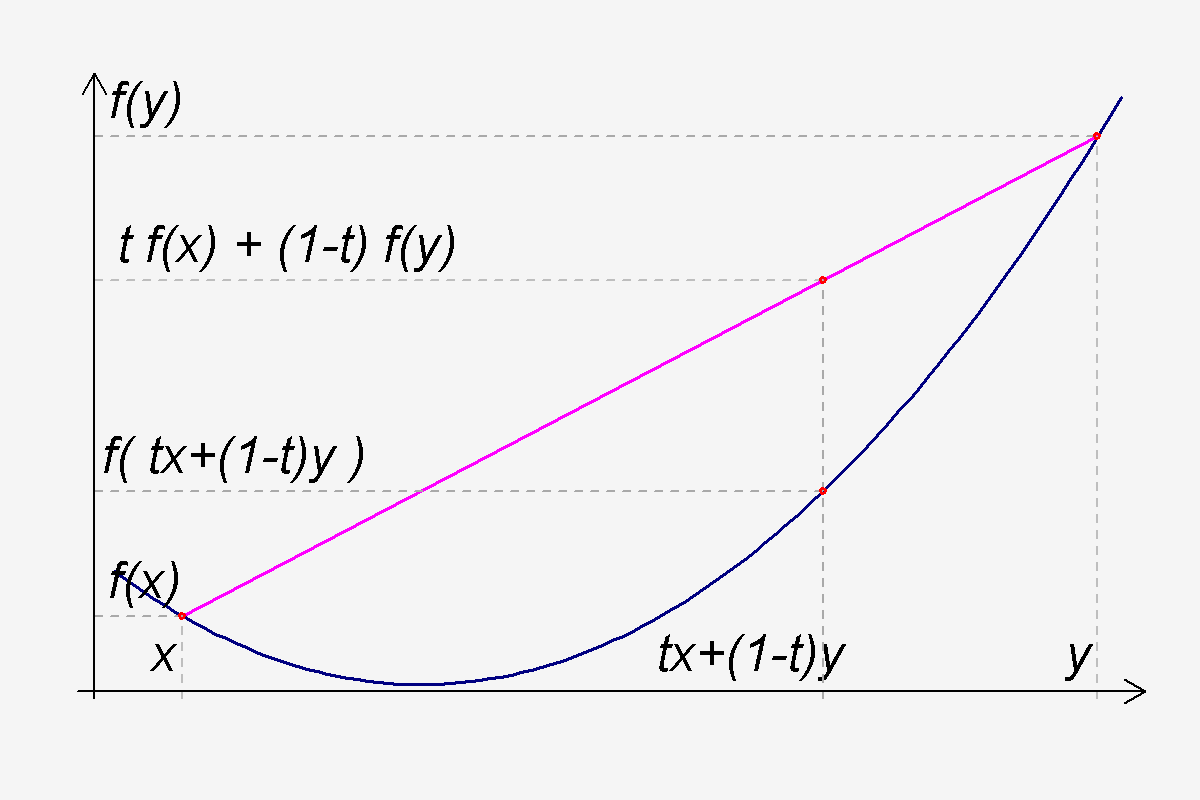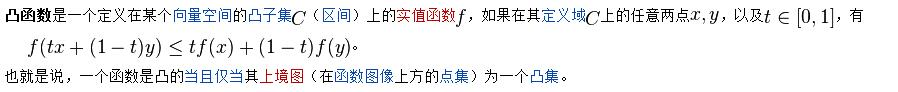注意：中国大陆数学界某些机构关于函数凹凸性定义和国外的定义是相反的。Convex Function在某些中国大陆的数学书中指凹函数。Concave Function指凸函数。但在中国大陆涉及经济学的很多书中，凹凸性的提法和其他国家的提法是一致的，也就是和数学教材是反的。举个例子，同济大学高等数学教材对函数的凹凸性定义与本条目相反，本条目的凹凸性是指其上方图是凹集或凸集，而同济大学高等数学教材则是指其下方图是凹集或凸集，两者定义正好相反。
为什么要求是凸函数呢？因为如果是下图这样的函数，则无法获得全局最优解。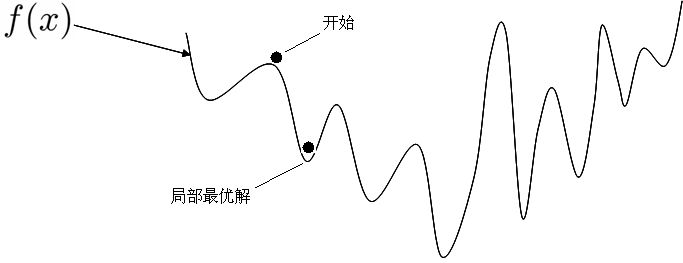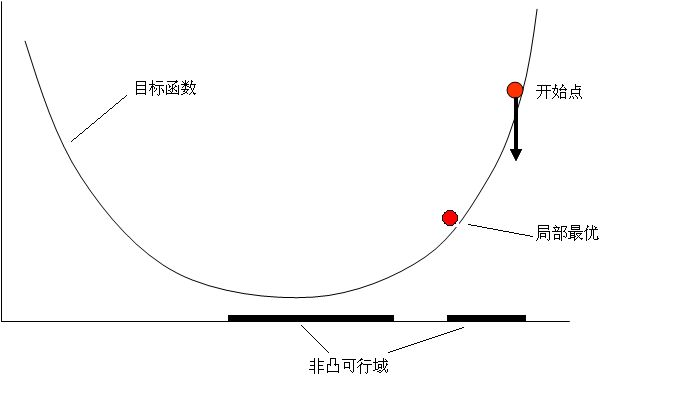为什么要求是凸集呢？因为如果可行域不是凸集，也会导致局部最优实际建模中判断一个最优化问题是不是凸优化问题一般看以下几点：

目标函数如果不是凸函数，则不是凸优化问题
决策变量中包含离散变量（0-1变量或整数变量），则不是凸优化问题
约束条件写成时，如果不是凸函数，则不是凸优化问题
之所以要区分凸优化问题和非凸的问题原因在于凸优化问题中局部最优解同时也是全局最优解，这个特性使凸优化问题在一定意义上更易于解决，而一般的非凸最优化问题相比之下更难解决。

非凸优化问题如何转化为凸优化问题的方法：

1）修改目标函数，使之转化为凸函数

2）抛弃一些约束条件，使新的可行域为凸集并且包含原可行域


展开全文算法 机器学习
• ## 深度学习: 凸 & 非凸 区别

万次阅读 多人点赞 2017-12-19 09:06:55
凸： 指的是顺着梯度方向走到底就 一定是 最优解 。 大部分 传统机器学习 问题 是凸的。 非凸： 指的是顺着梯度方向走到底只能保证是局部最优，不能保证 是全局...深度学习以及小部分传统机器学习问题都是非凸的。
凸：

指的是顺着梯度方向走到底就 一定是 最优解 。
大部分 传统机器学习 问题 都是凸的。
非凸：

指的是顺着梯度方向走到底只能保证是局部最优，不能保证 是全局最优。
深度学习以及小部分传统机器学习问题都是非凸的。

展开全文深度学习
• 深度学习算法的目标函数，几乎全都是非凸的。而目前寻找最优解的方法，是基于梯度下降的。稍微有点背景知识的人知道，梯度下降方法不能解决是解决非凸问题的。因此，如果找到最优解，将是深度学习领域，非常值得...
 1、局部最优问题。深度学习算法的目标函数，几乎全都是非凸的。而目前寻找最优解的方法，都是基于梯度下降的。稍微有点背景知识的人都知道，梯度下降方法不能解决是解决非凸问题的。因此，如果找到最优解，将是深度学习领域，非常值得研究的课题。andrew在google的工作，也就是那只猫，其实训练过程是让人很费解的。为了缩短训练时间，项目组采用了分布式训练的方式。采用了1000 台计算机，在不同的计算机上存储不同的训练数据，不同的训练服务器通过参数服务器进行参数的交换。训练过程开始后，所有的训练计算机从参数服务器更新当前 参数，然后利用当前参数以及本机器上的训练数据，计算得到当前的梯度，通过贪婪式方法，训练到不能再训练为止，然后将参数的更新量提交给服务器，再获取新 的参数进行更新。在这个过程中，出现了不同机器在同步时间上的一个大问题。具体阐述如下：梯度下降这种方法，在计算梯度的时候，一定要知道当前参数的具体值，梯度是 针对某一个具体的参数值才有意义的。但是，由于在这个系统中，计算机非常多，当计算机A从服务器上获得参数值后，完成梯度的计算得到步进量的时候，可能在 它提交结果之前，计算机B已经修改了参数服务器上的参数了。也就是说，A所得到的步进量，并不是针对当前的参数值的。论文中，作者注意到了这个问题，但是故意不去理会，结果训练结果居然不错。作者的解释是：这是一种歪打正着的现象。 为什么能够歪打正着呢？有可能是这样的：非凸问题，本来就不是梯度下降法能够解决的。如果不存在同步难题，那么随着训练的深入，结果肯定会收敛到某一个局部最优解上面去。而现在这种同步问题，恰好能够有助于跳出局部最优解。因此最终的训练结果还算不错。作者并没有证明，这种方式，对于寻找全局最优一定是有帮助的。对于最终的结果是否一定是经验最优的，也没有证明。因此我感觉，深度学习里面，这种超高维参数的最优结果的寻优，是一个很值得深入研究的问题。它对于最终的效果也确实影响很大。2、内存消耗巨大，计算复杂。 内存消耗巨大和计算复杂体现在两个方面。（1）训练过程。（2）检测过程。 这两个过程的计算复杂，根本原因都是庞大的参数规模造成的。比如google的这个项目，每一个位置都用到了8个模版，每一个像素，这8个模版都是 不同的，因此导致最后的模版总数很大，所以训练和检测都很慢。当然，这种模版的设计法，让人不好理解，为什么不同的像素位置，模版完全不同。我还是支持以 前的卷积神经网络里面的思想，不同位置的模版都是一样的，但没一个位置，模版数量就远不止8个了。这样的好处是，内存空间中，总的模板数下降了；但缺点 是，计算更复杂了。因此，如果能够找到一个好的方法，能够有效的较低计算复杂度，将是很有意义的。（比如某个邻域内如果方差极小，其实根本就没必要计算了，直接赋0.） 3、人脑机理还有很多没用上。 深度学习模拟的是人脑的其中一个很小的方面，就是：深度结构，以及稀疏性。 但事实上，人脑是相当复杂滴。关于视觉注意机制、多分辨率特性、联想、心理暗示等功能，目前根本就没有太多的模拟。所以神经解剖学对于人工智能的影响应该是蛮大的。将来要想掀起机器智能的另一个研究高潮，估计还得继续借鉴神经解剖学。4、人为设计模版的可行性。 一直在想，为什么第一层用于检测角点和边缘这种简单特征的模版，一定需要通过无监督训练得到，如果人为实现模拟的话，能否也得到较为理想的结果呢？ 从神经解剖学的成果上来看，人脑的v1区和v2区，神经细胞确实是按照规律排列的。而且都是可以人为设计的。而且，一个让人怀疑的地方就是，v1区和v2区的神经细胞，是先天发育好的，还是后天训练出来的？如果是先天的，那就是说，这种模版是可以人为设计的。5、代价函数的设计方法。 代价函数的设计，在初学者看来，是很奇怪的。代价函数的设计，直接影响到最终的模版训练结果，可以说是深度学习中最核心的模块。 从目前已经发表的论文来看，一是考虑重构误差，二是加入某种惩罚项。惩罚项的设计有多种模式，有考虑一阶范式的，有考虑二阶范式的，各种设计可谓千 奇百怪。有博文上讲到，惩罚项的作用是为了防止过拟合，但也有博文的观点是，惩罚项是为了保证稀疏性。（感觉过拟合与稀疏性是否存在某种内在联系。）当然，代价函数的设计方法，目前还在不断探索，感觉这是一个可以发论文的点。 6、整个神经网络系统的设计。 神经网络的设计方法，包含了研究人员对人脑的理解方式。CNN、RBM、RNN，以及andrew项目组设计的变态网络，都各有各的特色。要把整个网络框架设计好，还是比较需要经验的，也是相当费脑力的。当然，这是整个领域最有研究价值的模块
展开全文• 在实际工程问题中，比如现在我们用的最多的深度神经网络的求解优化问题都是非凸的，因此很多凸优化理论中非常有价值的定理和方法，在非凸优化问题中不适用，或者说并没有收敛保证等。但是，作为知识的基础，依然有...
凸优化理论本身非常博大，事实上我也只是了解了一个皮毛中的皮毛，但是对于广大仅仅想要了解一下机器学习或者深度学习的同学来说，稍微了解一点凸优化也就够了。在实际工程问题中，比如现在我们用的最多的深度神经网络的求解优化问题，都是非凸的，因此很多凸优化理论中非常有价值的定理和方法，在非凸优化问题中不适用，或者说并没有收敛保证等。但是，作为知识的基础，依然有必要来理解和学习一下凸优化，本篇整理了非常基础的一些概念。主要参考网上一些资料，和《凸优化》这本书的一些概念。
凸优化理论基础中的基础
1. 为什么要学习凸优化？
凸优化在数学规划领域具有非常重要的地位。一旦将一个实际问题表述为凸优化问题，大体上意味着相应问题已经得到彻底解决，这是非凸的优化问题所不具有的性质。其应用非常广泛，机器学习中很多优化问题都要通过凸优化来求解；在非凸优化中，凸优化同样起到重要的作用，很多非凸优化问题，可以转化为凸优化问题来解决；
2. 什么是优化问题？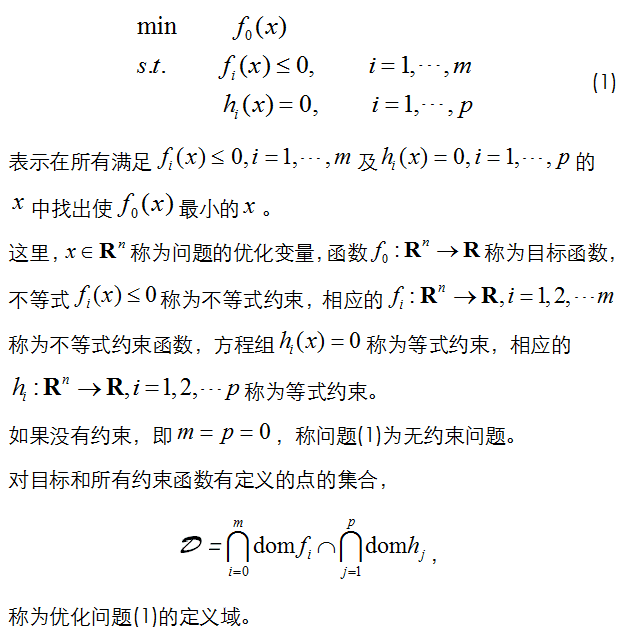3. 什么是凸优化问题？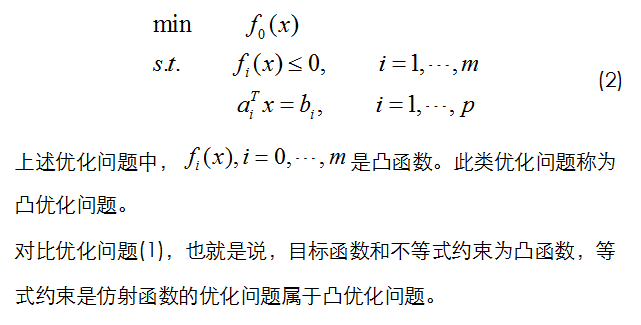4. 什么是凸函数？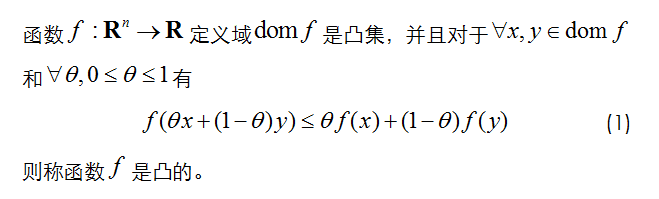有一个经典的示意图：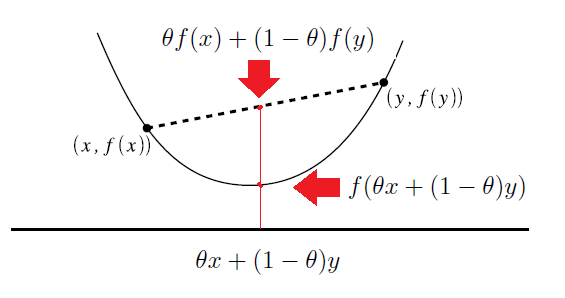凸函数的几何意义表示为函数任意两点的连线上的取值大于该点在函数上的取值
5. 凸函数的一阶充要条件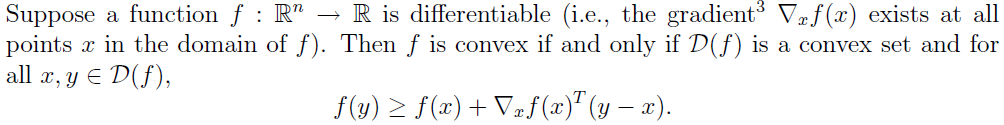一阶条件的意义是，对于函数在定义域的任意取值，函数的值都大于或者等于对函数在这点的一阶近似。用图来说明就是下图：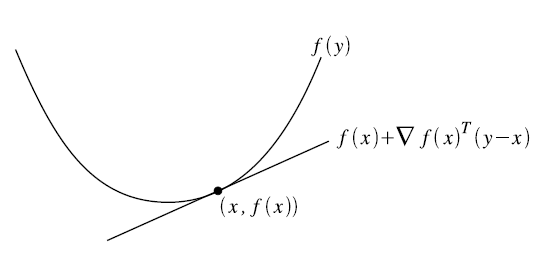口语化理解就是说凸函数总是在其任意一点的切线的上方（或者可以有部分相切的）。通过图可以很清楚地理解这个充要条件，但是，具体在应用中，我们不可能对每一个点都去计算函数的一阶导数，因此后面会说道利用凸函数的二阶特征来进行判断一个函数是否是一个凸函数。下面贴一下证明充分性和必要性的过程，来自《convex optimization》，不是很在意的同学可以跳过，直接看下一节。但是建议手推一下，还是比较简单的：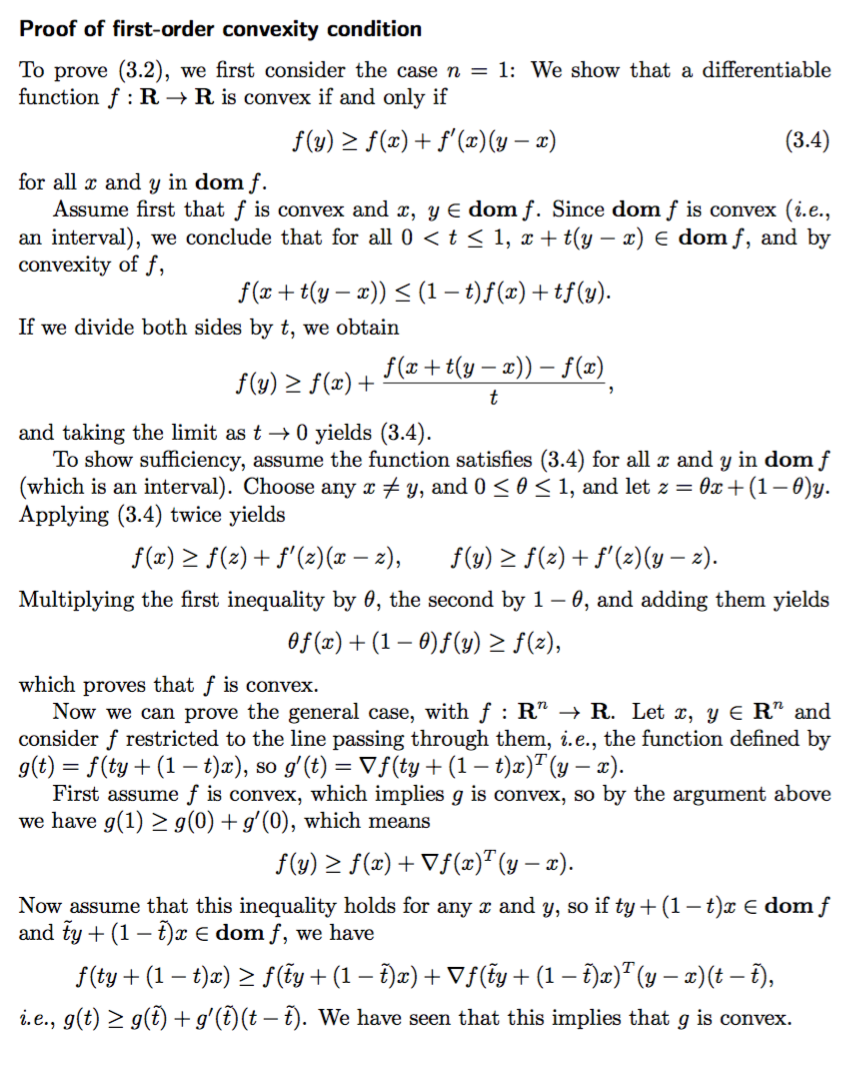6. 凸函数的二阶充要条件
记函数的一阶导数和二阶导数为g和H，
$g = \nabla f = \begin{bmatrix} \frac{\partial f }{\partial x_1} \\ \frac{\partial f }{\partial x_2} \\ \vdots\\\frac{\partial f }{\partial x_n} \\ \end{bmatrix} \ \ \ \ \quad H = \nabla^2 f = \begin{bmatrix} \frac{\partial^2 f }{\partial^2 x_1}& \frac{\partial^2 f }{\partial x_1 x_2} & \cdots &\frac{\partial ^2 f }{\partial x_1x_n} \\ \frac{\partial^2 f }{\partial^2 x_2x_1}& \frac{\partial^2 f }{\partial x_2^2} &\cdots &\frac{\partial ^2 f }{\partial x_2x_n} \\ \vdots & \vdots & \ddots & \vdots \\ \frac{\partial^2 f }{\partial^2 x_nx_1}& \frac{\partial^2 f }{\partial x_n x_2} &\cdots &\frac{\partial ^2 f }{\partial x_n^2} \\ \end{bmatrix}$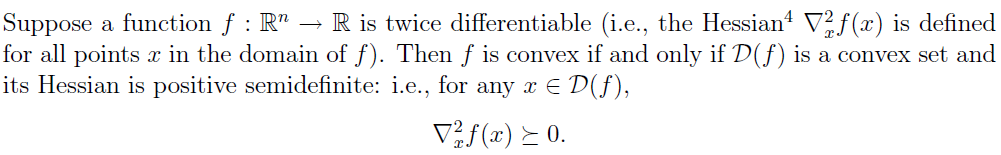其中要求函数$f$二阶可微，则函数$f$在定义域上式convex函数的充要条件是：函数的二阶导数（即Hessian矩阵）是半正定的，也就是所有的特征值都是大于等于0的。特殊的，对于一元函数，可以退化为$f''(x) \geq 0$。
凸函数举例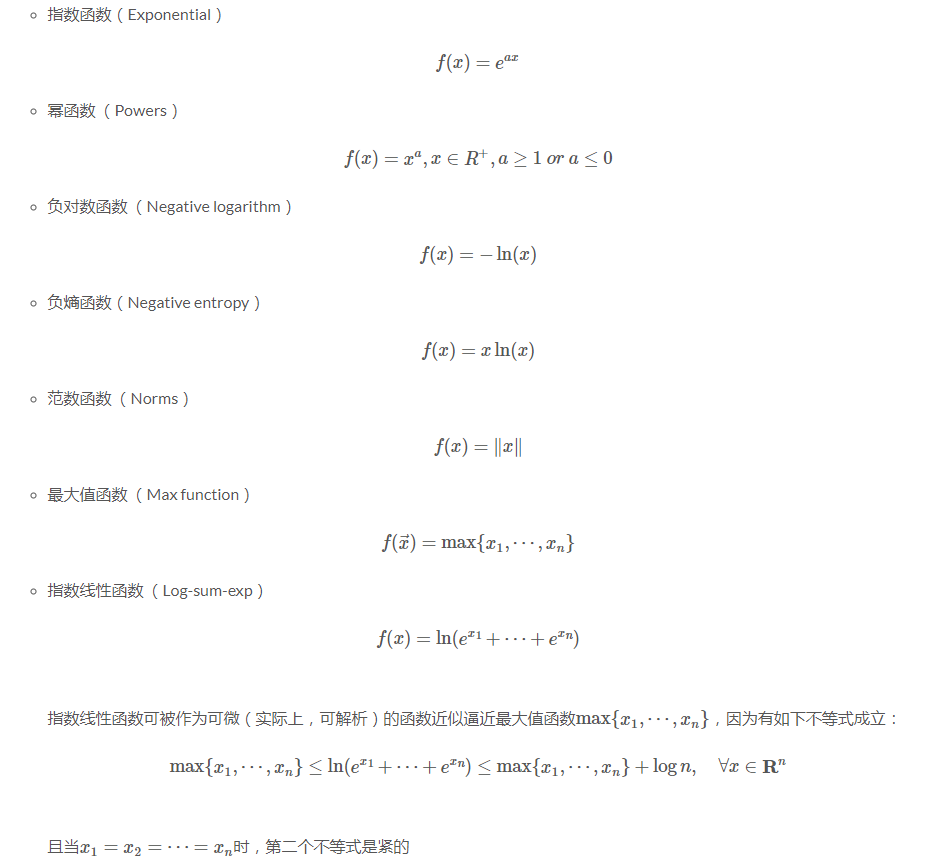举例用二阶充要条件来证明log-sum-exp函数是convex的：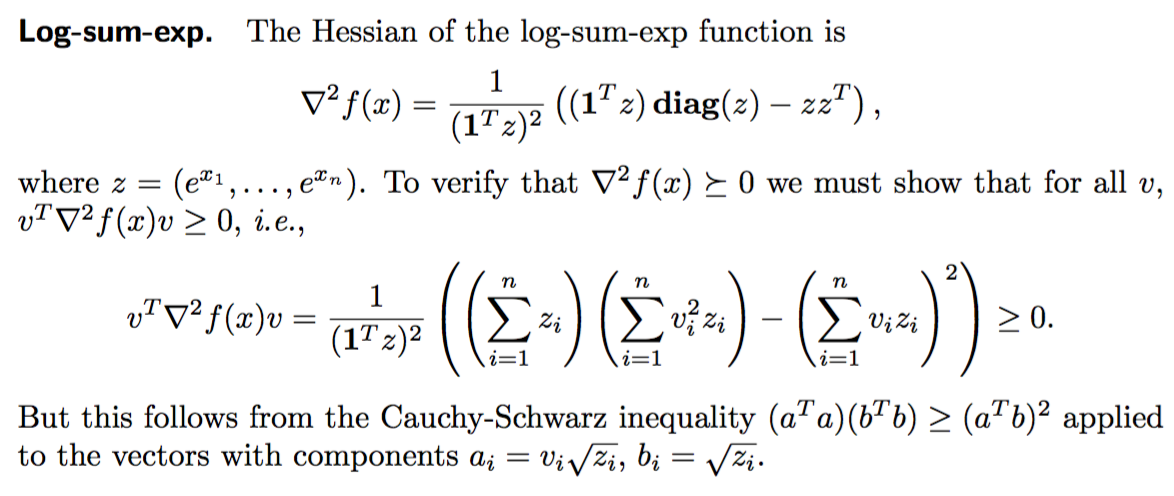最后一步用到了柯西不等式，很巧妙。

Hessian矩阵与牛顿法
上面提到了函数的二阶导数是Hessian矩阵，Hessian矩阵经常用于牛顿法优化方法中。牛顿法是一种迭代求解方法，有一阶和二阶方法，主要应用在两个方面：1、求方程的根， 2、 最优化方法。
（1）牛顿迭代求解方程的根：
并不是所有的方程都有求根公式, 或者求根公式很复杂, 导致求解困难. 利用牛顿法, 可以迭代求解。原理是利用泰勒公式, 在$x_0$处展开, 且展开到一阶, $x = x_0 + \delta$
$f(x) = f({x_0}) + (x – {x_0})f’({x_0})$
求解方程$f(x) = 0$，即$f({x_0}) + (x – {x_0})f’({x_0}) = 0$，整理后得到
$x = {x_0} – f({x_0})/f’({x_0})$
但是因为我们用的是一阶泰勒展开，只能近似相等，求得的解并不能完全使$f(x) = 0$成立。记这个金近似解为$x_1$，只能说$f(x_1)$比$f(x_0)$更接近$f(x) = 0$的解，因此我们可以写成一个递推公式：
${x_{k + 1}} = {x_k} – f({x_k})/f’({x_k}) \tag{3}$
通过迭代, 这个式子必然在$f(x^*)=0$的时候收敛。 整个过程如下图：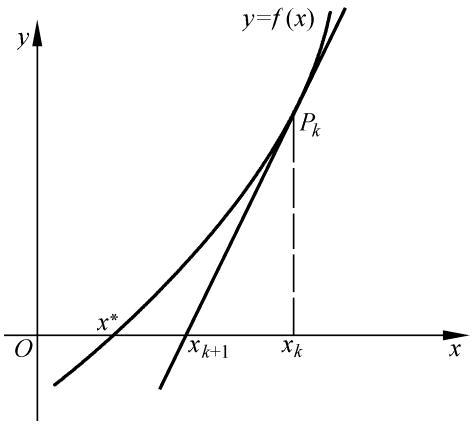一个比较形象的动态图：从初始点开始每次找f(x)的切线和坐标轴的交点，慢慢逼近真正的f(x)=0点。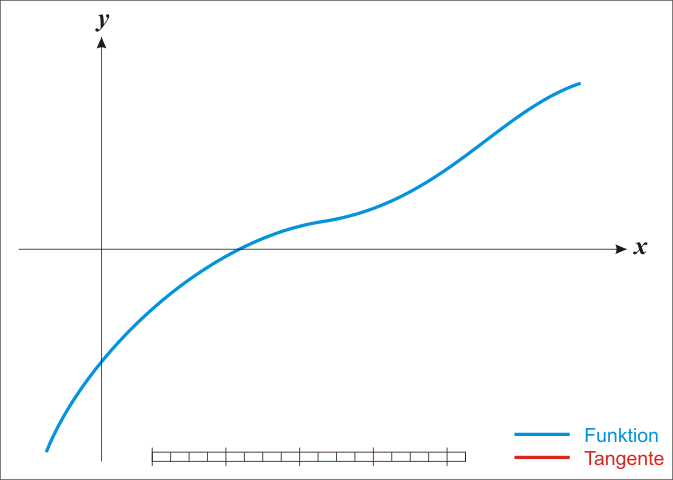（2）二阶优化方法——牛顿法
对于非线性优化问题，牛顿法提供了一种求解的办法。假设任务是优化一个目标函数$f(x)$, 求函数$f(x)$的极大或者极小问题, 可以转化为求解函数的导数$f'(x)=0$的问题, 这样求可以把优化问题看成方程求解问题。剩下的问题就和第一部分提到的牛顿迭代法求解很相似了。
我们先直接套用一下前面公式(3)，但是现在我们要把$f(x)$想象成$f'(x)$，要求$f'(x)=0$的根，则：
${x_{n + 1}} = {x_n} – \frac{f'({x_n})}{f''({x_n})}$
当然，拓展一下思维，我们也必然可以从泰勒展开获得推导：泰勒展开到二阶：
$f(x + \Delta x) = f\left( x \right) + f'(x)\Delta x + \frac{1}{2}f''(x)\Delta {x^2}$挪一下位置得到：
$f(x + \Delta x) - f\left( x \right) = f'(x)\Delta x + \frac{1}{2}f''(x)\Delta {x^2}$
假设我们要求$f(x)$的极小值（注：我们并没有假设$f(x)$是convex的，所以可能是一个局部极小值。如果有一定基础的同学，可以把$f(x)$理解为loss function），并且希望一步就要走到尽可能使$f(x)$变小最多（loss尽可能低），那么记，
$F= f'(x)\Delta x + \frac{1}{2}f''(x)\Delta {x^2} \leq 0$，并且绝对值最大，所以就是求F最小值。那就容易了，回到了初中数学~~~~，注意把$\Delta x$看成是待求变量，F对$\Delta x$求导等于0，得到
$\Delta x{\rm{ = - }}\frac{{f'({x})}}{{f''({x})}}$
得出牛顿法迭代公式：
${x_{n + 1}} = {x_n}{\rm{ - }}\frac{{f'({x_n})}}{{f''({x_n})}},n = 0,1,...$
（这里稍微扯一下一阶方法梯度下降，其实在上一篇中我们已经提到了，这里再强化啰嗦一次，如果只泰勒展开到一阶，那么上面的$f(x + \Delta x) - f\left( x \right)= f'(x)\Delta x \leq 0$，并且我们要求$f'(x)\Delta x$的最小值，$\Delta x$的模如果是一个常数，那么它的方向是$- f('x)$的方向时，取到最小值。所以梯度下降的公式就是： $x_{n + 1} = x_n - \eta \cdot f'(x)$。其中$\eta$是步长，表示每次沿着负梯度方向走多远。）
（2020年7月4日补充：上面的推导是可以的，另外一般的资料里往往直接从二阶泰勒展开求，这里也备注一下：假设任务是优化一个目标函数f, 求函数f的极大极小问题, 可以转化为求解函数f的导数$f^′=0$的问题。这次为了求解$f^′=0$的根, 首先把$f(x)$在探索点$x_n$处二阶泰勒展开：
$f(x) = f({x_n}) + f'({x_n})(x – {x_n}) + \frac{{f''({x_n})}}{2}{(x – {x_n})^2}$
$f(x)$对$x$求导数=0，则（注意$f(x_n)$这一项对$x$求导为0，）：
$f'(x) = f'({x_n}) + f''({x_n})(x – {x_n}) = 0$
整理得到迭代公式：
${x_{n + 1}} = {x_n}{\rm{ – }}\frac{{f'({x_n})}}{{f''({x_n})}},n = 0,1,…$
）
对于高维函数，牛顿法通用公式写成：
${x_{n + 1}} = {x_n} - {[Hf({x_n})]^{ – 1}}\nabla f({x_n}),n \ge 0$
一般认为牛顿法可以利用到曲线本身的信息, 比梯度下降法更容易收敛（迭代更少次数）, 如下图是一个最小化一个目标方程的例子, 红色曲线是利用牛顿法迭代求解, 绿色曲线是利用梯度下降法求解：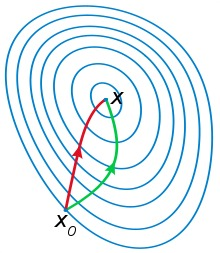牛顿法在多变量问题上仍然适用迭代求解，但Hessian矩阵的引入增加了复杂性，特别是当：
▪ Hessian 矩阵非正定，导致目标函数不一定下降，从而牛顿法不收敛；
▪ Hessian 矩阵维度过大带来巨大的计算量。
针对这个问题，在牛顿法无法有效执行的情况下，提出了很多改进方法，比如 拟牛顿法（Quasi-Newton Methods）可以看作是牛顿法的近似。拟牛顿法只需要用到一阶导数，不需要计算Hessian矩阵 以及逆矩阵，因此能够更快收敛，关于拟牛顿法这里不再具体展开，也有更深入的 DFP、BFGS、L-BFGS等算法，大家可以自行搜索学习。总体来讲，拟牛顿法都是用来解决牛顿法本身的复杂计算、难以收敛、局部最小值等问题。

OK，到目前写的几篇深度学习/机器学习入门基础数学知识整理基本涵盖了最最简单的线性代数，矩阵，泰勒展开，凸优化，梯度下降，牛顿法等，如果这些概念你都差不多清楚了，那么就开始学习深度学习或者机器学习吧，可以参考我的机器学习方法系列，以及深度学习方法系列，详见——我的Blog文章索引：：机器学习方法系列，深度学习方法系列，三十分钟理解系列等

看了"为什么最速下降法中迭代方向是锯齿形的"   ，补充一下。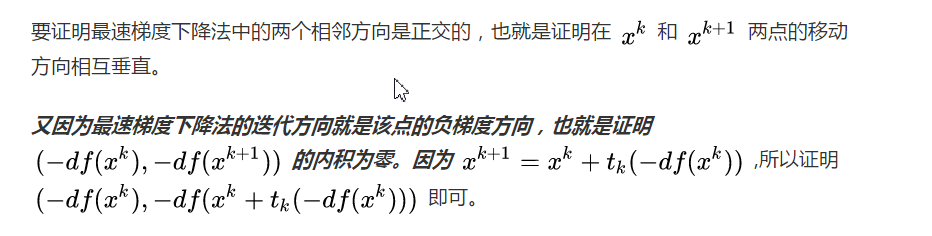最速梯度下降法的算法步骤，如下：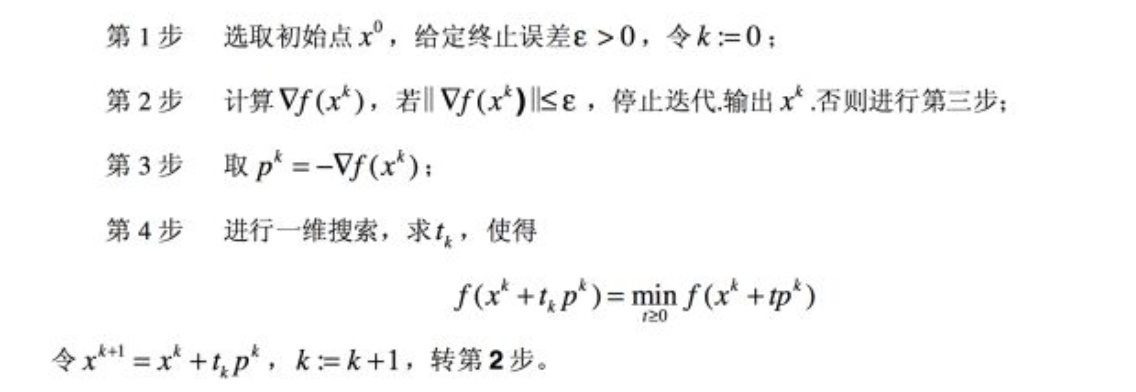最速梯度下降法中，我们在 $x^{k}$ 移动到 $x^{k+1}$ 的过程中，是对移动步长 $t_{k}$ 进行一维搜索，找到最优的 $x^{k+1}$ 。下面说明一下
对tk进行一维搜索，要满足
$\frac{\partial f(x^k -t_k \nabla f(x^k))}{\partial t_k} = \nabla f(x^k -t_k \nabla f(x^k))\cdot (-\nabla f(x^k)) = \nabla f(x^{k+1})\cdot (-\nabla f(x^k))=0$
可以发现，连续两次梯度方向是垂直的，也就是说最速梯度下降方法中连续两次更新方向是垂直。
参考资料
 《凸优化》(Stephen Boyd等著，王书宁等译)
 凸优化(一)——概述 https://www.jianshu.com/p/fe2e7f0e89e5
 【最优化】通俗理解凸函数及它的一阶特征 http://www.sohu.com/a/216564018_206784
 凸优化简介 http://www.cnblogs.com/ooon/p/5726238.html
 无约束优化方法(梯度法-牛顿法-BFGS- L-BFGS）http://www.cnblogs.com/ooon/p/5729982.html
 Jacobian矩阵和Hessian矩阵http://jacoxu.com/jacobian%E7%9F%A9%E9%98%B5%E5%92%8Chessian%E7%9F%A9%E9%98%B5/
 牛顿法与Hessian矩阵
http://blog.csdn.net/linolzhang/article/details/60151623
 https://zhuanlan.zhihu.com/p/33428233
 https://blog.csdn.net/batuwuhanpei/article/details/51979831
 凸优化（二）：凸函数的基本概念
 最优化学习笔记（四）——最速下降法


展开全文机器学习
• 一、单项选择题   1. 考察SVM 分类和深度... SVM 是凸问题深度学习都是非凸问题 正确，深度学习算法的目标函数，几乎全都是非凸的。   2. 考察 L1 和 L2 正则化的区别   L0 范数：向量中非0元素...
• Q：为什么深度学习损失函数往往要求是凸函数？（此处凸函数是指下凸，国内和国外凸函数的标准不一样，是相反的，吴恩达的机器学习教程凸函数是指下凸） A：使用梯度下降法优化策略，如果损失函数是非凸的，最后...机器学习 深度学习
• 一、单项选择题 ... SVM 是凸问题深度学习都是非凸问题 正确，深度学习算法的目标函数，几乎全都是非凸的。 L1L1L_1 和 L2L2L_2 正则化区别 L0L0L_0 范数：向量中非0元素的个数。 L1L1L_1...机器学习
• 美国艾尔弗·斯隆基金会（The Alfred P. Sloan Foundation）公布了2019年...他在个人主页上写道“深度学习等现代机器学习算法尝试从数据中自动学习有用的隐含表示。那么我们要如何公式化数据中的隐含结构，以及如...
• ## 深度学习常见算法的介绍和比较

万次阅读 多人点赞 2018-02-08 22:00:06
很多人有误解，以为深度学习比机器学习先进。其实深度学习是机器学习的一个分支。可以理解为具有多层结构的模型。具体的话，深度学习是机器学习中的具有深层结构的神经网络算法，即机器学习>神经网络算法>深度神经...深度学习
• 然而在训练神经网络时，我们遇到的问题大多是非凸，这就给优化带来更大的挑战。 3.1 局部极小值 凸优化问题通常可以简化为寻找一个局部极小值点的问题，在凸函数中，任何一个局部极小点是全局最小点；有些凸函数...
• 深度神经网络是一个高度非线性的模型，其风险函数是一个非凸函数，（因此风险最小化即深度学习的优化问题是非凸优化问题。）找到全局最优解比较困难。绝大多数深度学习中的目标函数很复杂。因此，很多优化问题并不...深度学习 优化问题
• ## 深度学习入门基础讲义

千次阅读 多人点赞 2017-11-28 16:22:35
工作确定以后，闲暇时间做了如下一个PPT讲义，用于向实验室新生学弟学妹介绍深度学习。他们大部分在本科期间学习通信相关专业课程，基本没有接触过图像处理和机器学习。对于一个研究生而言，自学应当是一个最基本也...深度学习
• 深度学习算法的目标函数，几乎全都是非凸的。而目前寻找最优解的方法，是基于梯度下降的。稍微有点背景知识的人知道，梯度下降方法是解决不了非凸问题的。因此，如果找到最优解，将是深度学习领域，非常值得研究...深度学习 大数据
• ## 深度学习概述

万次阅读 多人点赞 2016-03-06 20:59:05
算是一个科普文吧，文章中去除了复杂的公式和图表，主要内容包括深度学习概念、国内外研究现状、深度学习模型结构、深度学习训练算法、深度学习的优点、深度学习已有的应用、深度学习存在的问题及未来研究方向、深度...神经网络
...